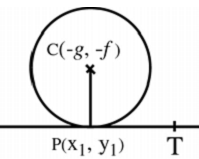# Equation of Tangent to a Circle

A tangent to a circle is a straight line which intersects (touches) the circle inexactly one point.

Let the equation of the circle be

x2 + y2 + 2gx + 2fy + c = 0

Let P(x1, y1) be a given point on it.

Let PT be the tangent at P.The centre of the circle is C(− g, − f).

How To Derive? Find slope of the CP. Since CP is perpendicular to PT, find slope of PT. Find equation of tangent in slope-intercept form.

The equation of the tangent at (x1, y1) is

xx1 + yy1 + g(x+x1) + f(y+y1) + c = 0

The equation of the tangent at (x1, y1) to the circle x2 + y2 = a2 is

xx1 + yy1 = a2